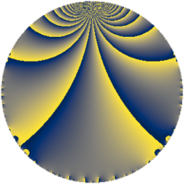Properties

 Label 6930.2.a.bpLevel $6930$ Weight $2$ Character orbit 6930.a Self dual yes Analytic conductor $55.336$ Analytic rank $1$ Dimension $2$ CM no Inner twists $1$

Related objects

Newspace parameters

 Level: $$N$$ $$=$$ $$6930 = 2 \cdot 3^{2} \cdot 5 \cdot 7 \cdot 11$$ Weight: $$k$$ $$=$$ $$2$$ Character orbit: $$[\chi]$$ $$=$$ 6930.a (trivial)

Newform invariants

 Self dual: yes Analytic conductor: $$55.3363286007$$ Analytic rank: $$1$$ Dimension: $$2$$ Coefficient field: $$\Q(\sqrt{13})$$ Defining polynomial: $$x^{2} - x - 3$$ Coefficient ring: $$\Z[a_1, \ldots, a_{13}]$$ Coefficient ring index: $$2$$ Twist minimal: yes Fricke sign: $$1$$ Sato-Tate group: $\mathrm{SU}(2)$

$q$-expansion

Coefficients of the $$q$$-expansion are expressed in terms of $$\beta = \sqrt{13}$$. We also show the integral $$q$$-expansion of the trace form.

 $$f(q)$$ $$=$$ $$q - q^{2} + q^{4} - q^{5} + q^{7} - q^{8} +O(q^{10})$$ $$q - q^{2} + q^{4} - q^{5} + q^{7} - q^{8} + q^{10} + q^{11} + ( 3 + \beta ) q^{13} - q^{14} + q^{16} + ( -1 - \beta ) q^{17} + 2 q^{19} - q^{20} - q^{22} + ( -1 - \beta ) q^{23} + q^{25} + ( -3 - \beta ) q^{26} + q^{28} -6 q^{29} -4 q^{31} - q^{32} + ( 1 + \beta ) q^{34} - q^{35} + ( -3 + \beta ) q^{37} -2 q^{38} + q^{40} + ( -4 + 2 \beta ) q^{41} -2 \beta q^{43} + q^{44} + ( 1 + \beta ) q^{46} + ( -4 + 2 \beta ) q^{47} + q^{49} - q^{50} + ( 3 + \beta ) q^{52} + ( -4 + 2 \beta ) q^{53} - q^{55} - q^{56} + 6 q^{58} + ( -6 - 2 \beta ) q^{61} + 4 q^{62} + q^{64} + ( -3 - \beta ) q^{65} + ( -1 - 3 \beta ) q^{67} + ( -1 - \beta ) q^{68} + q^{70} + ( 2 - 4 \beta ) q^{71} -10 q^{73} + ( 3 - \beta ) q^{74} + 2 q^{76} + q^{77} + ( 6 - 2 \beta ) q^{79} - q^{80} + ( 4 - 2 \beta ) q^{82} + ( 2 + 2 \beta ) q^{83} + ( 1 + \beta ) q^{85} + 2 \beta q^{86} - q^{88} + ( -5 + \beta ) q^{89} + ( 3 + \beta ) q^{91} + ( -1 - \beta ) q^{92} + ( 4 - 2 \beta ) q^{94} -2 q^{95} + ( 12 - 2 \beta ) q^{97} - q^{98} +O(q^{100})$$ $$\operatorname{Tr}(f)(q)$$ $$=$$ $$2 q - 2 q^{2} + 2 q^{4} - 2 q^{5} + 2 q^{7} - 2 q^{8} + O(q^{10})$$ $$2 q - 2 q^{2} + 2 q^{4} - 2 q^{5} + 2 q^{7} - 2 q^{8} + 2 q^{10} + 2 q^{11} + 6 q^{13} - 2 q^{14} + 2 q^{16} - 2 q^{17} + 4 q^{19} - 2 q^{20} - 2 q^{22} - 2 q^{23} + 2 q^{25} - 6 q^{26} + 2 q^{28} - 12 q^{29} - 8 q^{31} - 2 q^{32} + 2 q^{34} - 2 q^{35} - 6 q^{37} - 4 q^{38} + 2 q^{40} - 8 q^{41} + 2 q^{44} + 2 q^{46} - 8 q^{47} + 2 q^{49} - 2 q^{50} + 6 q^{52} - 8 q^{53} - 2 q^{55} - 2 q^{56} + 12 q^{58} - 12 q^{61} + 8 q^{62} + 2 q^{64} - 6 q^{65} - 2 q^{67} - 2 q^{68} + 2 q^{70} + 4 q^{71} - 20 q^{73} + 6 q^{74} + 4 q^{76} + 2 q^{77} + 12 q^{79} - 2 q^{80} + 8 q^{82} + 4 q^{83} + 2 q^{85} - 2 q^{88} - 10 q^{89} + 6 q^{91} - 2 q^{92} + 8 q^{94} - 4 q^{95} + 24 q^{97} - 2 q^{98} + O(q^{100})$$

Embeddings

For each embedding $$\iota_m$$ of the coefficient field, the values $$\iota_m(a_n)$$ are shown below.

For more information on an embedded modular form you can click on its label.

Label $$\iota_m(\nu)$$ $$a_{2}$$ $$a_{3}$$ $$a_{4}$$ $$a_{5}$$ $$a_{6}$$ $$a_{7}$$ $$a_{8}$$ $$a_{9}$$ $$a_{10}$$
1.1
 −1.30278 2.30278
−1.00000 0 1.00000 −1.00000 0 1.00000 −1.00000 0 1.00000
1.2 −1.00000 0 1.00000 −1.00000 0 1.00000 −1.00000 0 1.00000
 $$n$$: e.g. 2-40 or 990-1000 Significant digits: Format: Complex embeddings Normalized embeddings Satake parameters Satake angles

Atkin-Lehner signs

$$p$$ Sign
$$2$$ $$1$$
$$3$$ $$1$$
$$5$$ $$1$$
$$7$$ $$-1$$
$$11$$ $$-1$$

Inner twists

This newform does not admit any (nontrivial) inner twists.

Twists

By twisting character orbit
Char Parity Ord Mult Type Twist Min Dim
1.a even 1 1 trivial 6930.2.a.bp 2
3.b odd 2 1 6930.2.a.cc yes 2

By twisted newform orbit
Twist Min Dim Char Parity Ord Mult Type
6930.2.a.bp 2 1.a even 1 1 trivial
6930.2.a.cc yes 2 3.b odd 2 1

Hecke kernels

This newform subspace can be constructed as the intersection of the kernels of the following linear operators acting on $$S_{2}^{\mathrm{new}}(\Gamma_0(6930))$$:

 $$T_{13}^{2} - 6 T_{13} - 4$$ $$T_{17}^{2} + 2 T_{17} - 12$$ $$T_{19} - 2$$ $$T_{23}^{2} + 2 T_{23} - 12$$ $$T_{29} + 6$$ $$T_{31} + 4$$

Hecke characteristic polynomials

$p$ $F_p(T)$
$2$ $$( 1 + T )^{2}$$
$3$ $$T^{2}$$
$5$ $$( 1 + T )^{2}$$
$7$ $$( -1 + T )^{2}$$
$11$ $$( -1 + T )^{2}$$
$13$ $$-4 - 6 T + T^{2}$$
$17$ $$-12 + 2 T + T^{2}$$
$19$ $$( -2 + T )^{2}$$
$23$ $$-12 + 2 T + T^{2}$$
$29$ $$( 6 + T )^{2}$$
$31$ $$( 4 + T )^{2}$$
$37$ $$-4 + 6 T + T^{2}$$
$41$ $$-36 + 8 T + T^{2}$$
$43$ $$-52 + T^{2}$$
$47$ $$-36 + 8 T + T^{2}$$
$53$ $$-36 + 8 T + T^{2}$$
$59$ $$T^{2}$$
$61$ $$-16 + 12 T + T^{2}$$
$67$ $$-116 + 2 T + T^{2}$$
$71$ $$-204 - 4 T + T^{2}$$
$73$ $$( 10 + T )^{2}$$
$79$ $$-16 - 12 T + T^{2}$$
$83$ $$-48 - 4 T + T^{2}$$
$89$ $$12 + 10 T + T^{2}$$
$97$ $$92 - 24 T + T^{2}$$